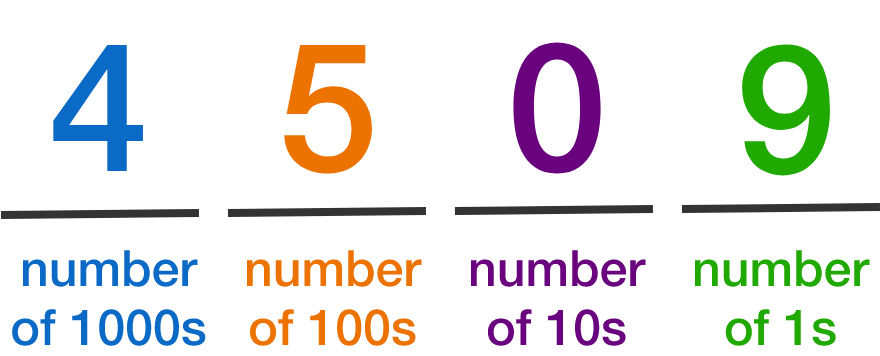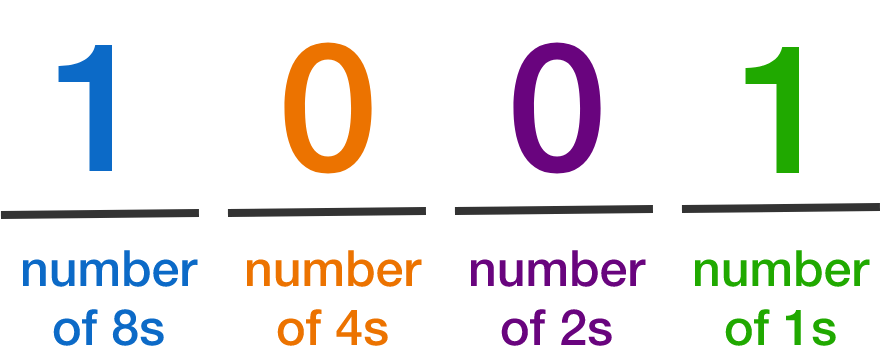### Number Bases

In our base-10 system, the positions (from right to left) correspond to "number of 1s", "number of 10s", "number of 100s", and so on, where the value of each position is 10 times the previous one.Binary, or base 2, uses only the digits 0 and 1. Each position corresponds to "number of 1s", "number of 2s", "number of 4s", "number of 8s", and so on. The value of each position is 2 times the previous one.The number above expressed in base 10 is $8 + 1 = 9 ,$ since there is one 8 and one 1.

What is the value (in base 10) of the binary number 10000?

# Introducing Binary

Which binary number is larger?

# Introducing Binary

If a binary number ends in 1 (like 1001 or 111011), then it is $\text{\_\_\_\_\_\_\_\_\_\_\_\_\_\_\_\_\_}.$

# Introducing Binary

What is the result (in binary) of multiplying the binary number 1100 by the binary number 10?

# Introducing Binary

Here are the binary numbers listed starting from 2. Consider the second from the last digit.

10, 11, 100, 101, 110, 111, 1000, 1001, 1010, 1011, 1100, 1101, 1110, 1111, ...

Notice the pattern 1, 1, 0, 0, 1, 1, 0, 0, 1, 1, 0, 0, ...

Does this pattern continue for all positive integers?

×